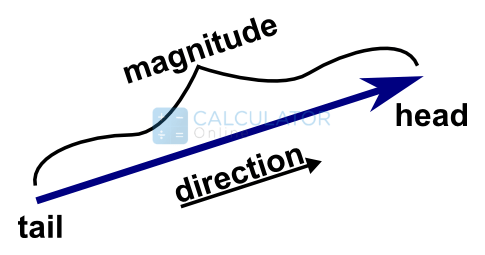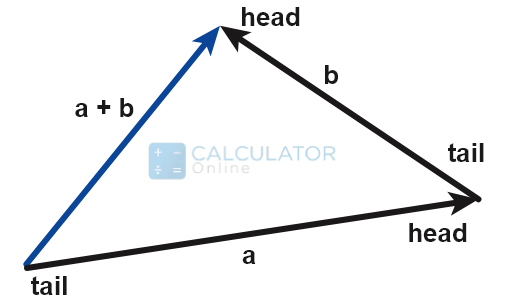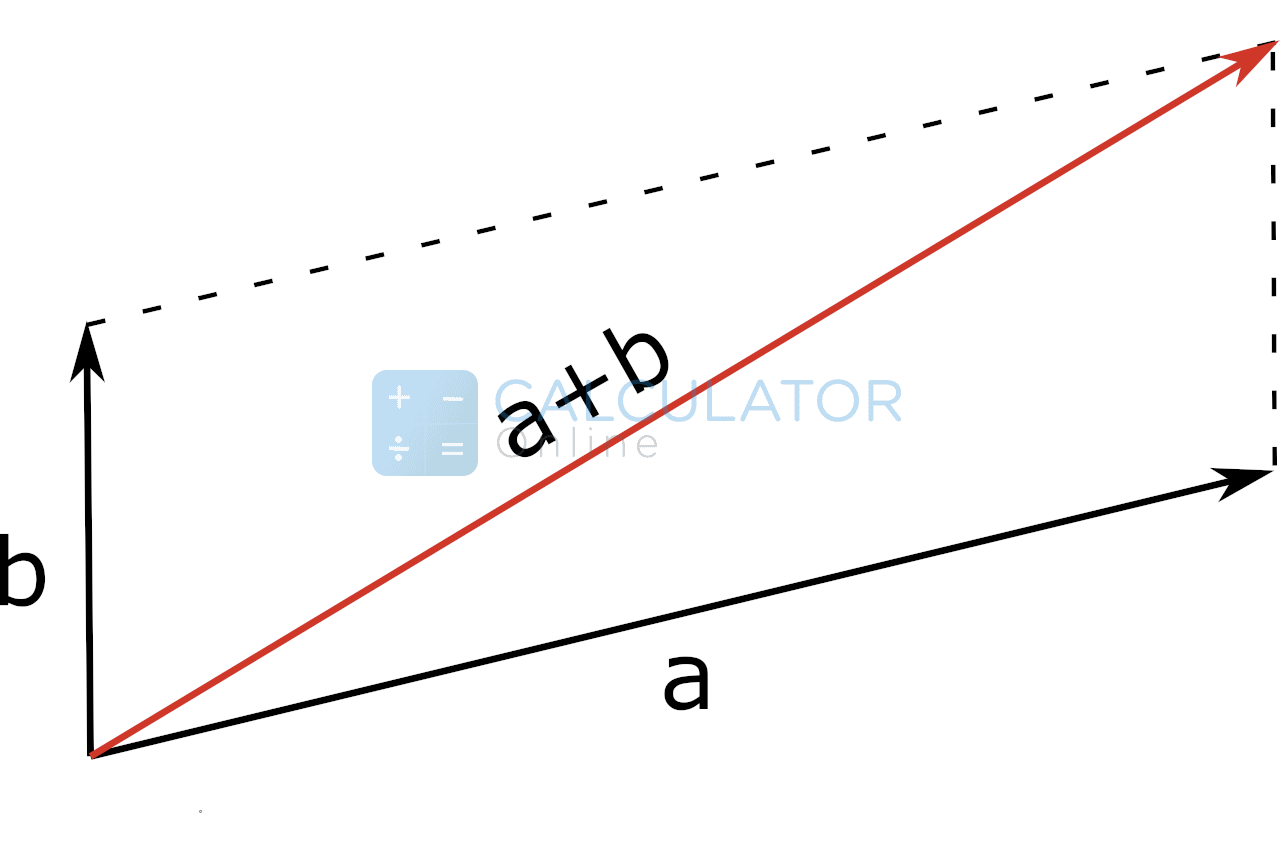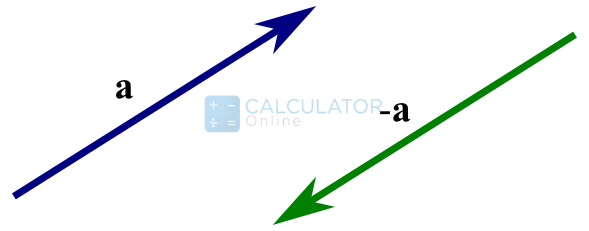•Sign In
• Hire UsUh Oh! It seems you’re using an Ad blocker!

We always struggled to serve you with the best online calculations, thus, there's a humble request to either disable the AD blocker or go with premium plans to use the AD-Free version for calculators.

OrDimension of a vector:

Operation

α

β

Vector A Representation

First Vector (a)

x

y

z

magnitude (m)

direction (θ)

Vector B Representation

First Vector (b)

x

y

z

magnitude (m)

direction (θ)

Table of Content

 1 What is priceeight Class? 2 priceeight Class Chart: 3 How to Calculate priceeight Density (Step by Step): 4 Factors that Determine priceeight Classification: 5 What is the purpose of priceeight Class? 6 Are mentioned priceeight Classes verified by the officials? 7 Are priceeight Classes of UPS and FedEx same?

Get the Widget!

Add this calculator to your site and lets users to perform easy calculations.

Feedback

How easy was it to use our calculator? Did you face any problem, tell us!

This free vector addition calculator allows you to calculate the sum of two vectors (with or without multiples) in a 2d and 3d coordinate system.

## What Is A Vector?

“A quantity that is represented by magnitude and direction is known as the vector”### Pictorial Representation:

In above picture:

• The straight line represents the magnitude of the vector
• Direction of the vector is denoted by the arrow head

Vectors are represented in terms of coordinates or multiples of coordinates. Following are vector sum formulas that are used to show vector combination as a whole. Let’s go through these!

#### Without Multiples:

##### 2D System:

(a,b) + (d,e) = (a + d, b + e)

##### 3D System:

(a,b,c) + (d,e,f) = (a + d, b + e, c + f)

Our free vector addition calculator also considers the formulas to add or subtract vectors accordingly.

#### With Multiples:

Now consider you come across multiples that help you create a sum of vectors more than twice. Just take an example:

Suppose you have the expression as follows:

a+b*2

The above expression means you are likely to add 2 image copies of b to a. With our free sum of vectors calculator, you can readily solve for vector addition or subtraction either with or without multiples.

This is another rule of vector addition that lets you count the sum of vectors without coordinates in general. Let’s throw a light at the rule first:

Consider you have two vectors a and b. To add them, join the tail of the vector b to the head of vector a. Now, take the resultant vector c such that its tail coincides with the tail of vector a and head with the head of vector b. This vector c represents the sum of two vectors that you could also determine with the aid of this online free to use vector ader

#### Pictorial Representation:### Parallelogram Rule:

Apart from the head to tail rule of vector addition, this is another rule and is considered more reliable than all. Now let us make it clear here that whatever the method or rule you opt for calculations, this free vector addition calculator will get it done for you in a couple seconds while maintaining accuracy. Also, you can also make use of our best sig fig calculator to get maximum accuracy for any calculation or number.

#### Statement:

If you wish to add two vectors a and b, join both of them such that their tails coincide with one another. Now create a dotted line just opposite in look at the vectors combined. At last, the diagonal of the parallelogram will represent the sum of vectors that you can also determine with the assistance of this free 2D vector addition calculator.

#### Pictorial Representation:### How To Subtract Vectors?

No doubt you can make use of the free vector subtraction calculator to minus one vector from the other, but here we will take you through the brief concept as well.Look at the above picture! What do you see? Let us explain!

We have two vectors a and -a, where a being the positive vector and -a being the negative vector.

“A negative vector is the one having same magnitude to the original vector but direction opposite to it”

Now when you want to subtract two vectors, it means you need to add the original vector to its opposite vector. For vectors a and -a, we have:

a-a = a+(a-)

You can also add two vectors easily by the aid of this subtracting vectors calculator.

### How To Add or Subtract Two Vectors?

Let’s resolve an example to understand the concept of vector sum or minus better!

Example # 01:

How to add vectors given as below:

Vector A = (1, 4)

Vector B = (6, 8)

Solution:

Vector A + Vector B = (1, 4) + (6, 8)

Vector A + Vector B = (1+6, 4+8)

Vector A + Vector B = (7, 14)

For instant verification, you may trust the calculations of our free vector adder.

### How Vector Addition Calculator Works?

Let’s get to know how you could use this add vectors calculator to perform addition and subtraction of two vectors with or without multiples.

Input:

• From the first drop-down list, select the dimension of vectors
• After that, select the type of addition or subtraction you want to perform(either with or without multiples)
• Now write down the coordinates of the vectors in their respective fields
• At last, hit the calculate button

Output:

The free adding vectors graphically calculator carries out the following calculations:

• Vector sum
• Vector subtraction
• The add vector calculator also displays step by step calculations to understand the solution better

## FAQ’s:

### How are vectors used in everyday life?

In real life, there are a huge number of vector applications. Just consider an example of a boat and its engine. Where the engine applies force in one particular direction to move the boat, the tides will apply an opposite force to the direction of the boat. And to understand the actual directions of these forces, you can use another  vector projection calculator to get precise outcomes.

### How important is the concept of vector in engineering?

In the field of engineering sciences, the vector has a significant theory indeed. It helps to find vector sum and subtraction for most of the physical or mechanical quantities such as force, work, torque etc. and here now, you can also use this free vector sum calculator to analyse the addition of such vector parameters in no time without compromising accuracy in results.

### What do you mean by the magnitude of the vector?

Simply, the magnitude of a vector is the length of the vector from the starting to the terminal point.

#### Representation:

If v is a any vector, then its magnitude is denoted by the following formula:

Vector Magnitude = |v|

Moreover, we have another vector magnitude calculator to determine the norm of a vector in a span of moments. Also, if you wish for adding magnitudes of vectors, you can also do that with the aid of this simple and online calculator.

## Conclusion:

The concept of vector addition and subtraction is highly significant and has applications in almost every sector of science and technology. And keeping in view the actual importance of the concept, we have designed this best online 2D vector addition calculator. Just inhibit your research and start using this adding vectors calculator to enjoy the exact and fast computations regarding your vector arithmetic.

## References:

From the source of Wikipedia: Euclidean vector, History, Cartesian space, affine vectors, Generalizations, Decomposition or resolution, Basic properties, Scalar multiplication, Scalar triple product, Conversion between multiple Cartesian bases

From the source of Khan Academy: Add vectors, subtracting vectors end-to-end, Magnitude

From the source of Lumen Learning: Graphical Methods, Vectors in Two Dimensions, Head-to-Tail Method, Vector Subtraction, Resolving a Vector into Components